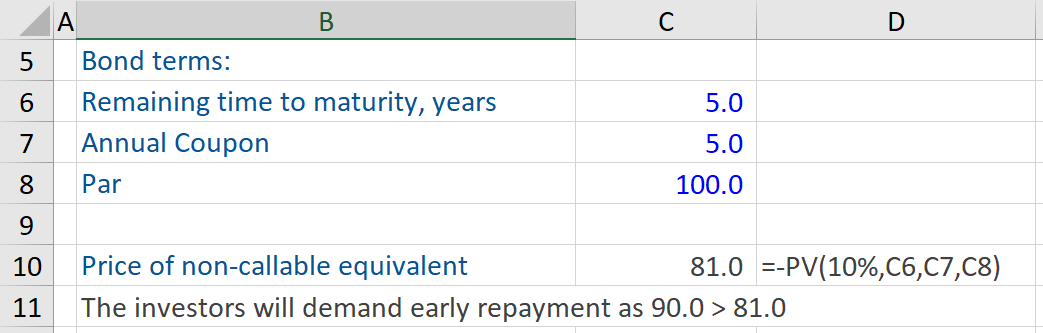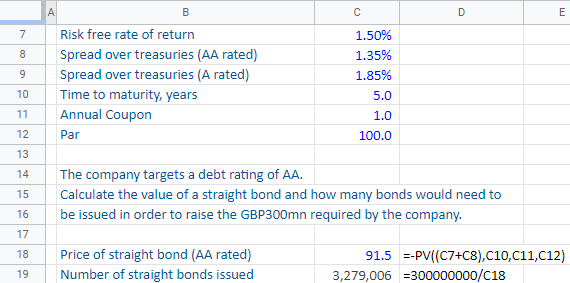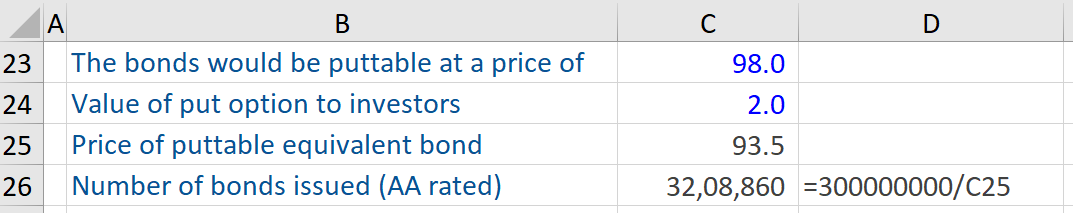## What are “Puttable Bonds”?

Puttable bonds are plain vanilla bonds, with an embedded put option. In other words, investors who are holders of this type of bond have the right but not the obligation to sell their bond back to the issuer at a specified price prior to the maturity date. In essence, puttable bonds can be sold back by the investor to the issuer from whom they had originally purchased it and get their money back.

In the case of puttable bonds, while the option to redeem before maturity is an advantage, such bonds offer a lower yield than regular bonds. However, their price is higher than identical conventional bonds. Furthermore, if the bondholder opts to exercise this right, then he or she will receive the principal value of the bond – which is paid at par value.

## Key Learning Points

• Puttable bonds are plain vanilla bonds with an embedded put option
• The price of a puttable bond is equal to the price of a conventional bond plus the value of the put option
• Puttable bonds offer a lower cost of borrowing to issuers and more flexibility for investors
• The maturity range of most puttable bonds is one to five years

## Characteristics of Puttable Bonds

Puttable bonds are the direct opposite of callable bonds, which are bonds that give the issuer right but not the obligation to purchase the bonds back from the investors prior to their maturity date.

Puttable bonds can be advantageous to both parties – the issuer and the investor. By issuing puttable bonds, the issuer can lower their borrowing costs. Investors are willing to pay a bit more for bonds with an embedded put option because it provides substantial flexibility to the investor vis-à-vis redeeming the bond. Investors can lower their reinvestment risk by investing in such bonds. In the event of an interest rate rise, investors in puttable bonds can exercise the option to sell these bonds which are trading at a lower coupon. Thereafter, they can reinvest the proceeds in purchasing other bonds in the market paying higher yields. Or, if after investing in a puttable bond, interest rates rise, which lower this bond’s value, the bondholder of such bonds can compel the issuer to redeem the bond at par value and partially or fully recover their loss.

Most puttable bonds have a maturity range of between one and five years and there are often multiple sell dates throughout the duration of a puttable bond.

## Puttable Bonds – Pricing Formula

The formula for pricing a puttable bond is given below. The formula shows that the pricing of a puttable bond is a combination of a straight or conventional bond and a put option:

Pb = Pc + VAp
where,
Pb = price of the putable bonds
Pc = price of conventional bonds (= present value of coupon payments and redemption value)
VAp = value of the put option

## Puttable Bonds Examples

The bond in the example below is puttable at \$90. It was issued when interest rates were very low. Rates have since increased significantly and the yield to maturity (YTM) for a non-puttable (straight) bond with the same terms is 10%.

As this is a puttable bond, the investor can sell it at a pre-defined price if he or she chooses to do so. The question is what will the investor in these bonds do? To determine the best course of action, the investor will calculate the price of a non-callable equivalent bond here. Taking the YTM of 10% and using the -PV function with 5 years remaining time to maturity, an annual coupon of 5%, and a par value of \$100 give a price of a non-callable equivalent bond of \$81.In this case, the investor can sell these bonds back at \$90 and buy other bonds with exactly the same terms other than not being puttable for \$81 and monetize the difference. A rational, logical investor will demand early repayment as the puttable price of \$90 is bigger than \$81.

The next example shows a scenario where a company would consider issuing puttable bonds. Company A has decided to raise GBP 300 million by issuing bonds. The bond and market terms are detailed below.The risk-free rate is 1.50%.

The spread over Treasuries for AA-rated bonds is 1.35% and 1.85% for single A-rated bonds.

The time to maturity is 5 years and the annual coupon is expected to be 1%, while the par value is \$100.

The company has decided to target a debt rating of AA. The first step would be to calculate the value of a straight bond and the number of bonds the company would need to issue in order to raise the GBP 300 million required by the company.

Using the -PV function in Excel and taking the price of the straight bond (AA rated), the risk-free rate of return of 1.50%, the spread over treasuries (for a AA rated entity) of 1.35%, number of periods (5 years), coupon (1.0) and a bond par value of \$100 gives a price of a straight bond (AA rated) of \$ 91.5. Therefore the company expects to raise \$91.5 per bond here. In order to raise GBP 300 million, the company needs to sell/issue 3,279,006 bonds ( GBP 300 million divided by \$91.5)

Now let us consider a puttable bond issue, rather than a straight bond issue. Let’s say we are going to make them puttable at \$98. Well, then they go out and figure out what the value of this put option is to investors and in this case, we have decided that the value of that put option is \$2. We assume that the value of the put option here for investors is \$2.Next, we know that a puttable bond is more valuable than a straight bond, so now we can figure out what the price of a puttable equivalent bond would be. It is simply going to be the price of the straight bond i.e. \$91.5 + the value of the put option (\$2) i.e. \$93.5. So, the price of the puttable equivalent bond is \$93.5.

Now, if the firm decides to issue puttable bonds, how many bonds does the firm have to issue?  We calculate this i.e. we take the number of bonds issued (AA rated) as GBP 300 million and divide it by the price per bond i.e. \$93.5, which is equal to 3,208,860. In other words, the firm would have to issue 3,208,860 bonds. So, significantly fewer bonds here if they decide to issue puttable bonds.

## Conclusion

Lastly, puttable bonds are more valuable than straight bonds and the difference there is the value of the put option to the investors. For issuing companies, puttable bonds are a great avenue to obtain debt financing for business operations, as they pay a lower rate of interest, while such bonds lower the reinvestment risk for investors.